## Example Questions

← Previous 1

### Example Question #1 : Nth Term Of An Arithmetic Sequence

2, 8, 14, 20

The first term in the sequence is 2, and each following term is determined by adding 6. What is the value of the 50th term?

296

320

300

302

296

Explanation:

We start by multiplying 6 times 46, since the first 4 terms are already listed. We then add the product, 276, to the last listed term, 20. This gives us our answer of 296.

### Example Question #11 : Arithmetic Sequences

Which of the following could not be a term in the sequence 5, 10, 15, 20...?

3751

2500

35

10005

3751

Explanation:

All answers in the sequence must end in a 5 or a 0.

### Example Question #1 : How To Find The Nth Term Of An Arithmetic Sequence

In an arithmetic sequence, each term is two greater than the one that precedes it. If the sum of the first five terms of the sequence is equal to the difference between the first and fifth terms, what is the tenth term of the sequence?

10

–0.6

2.4

15.6

0.6

15.6

Explanation:

Let a1 represent the first term of the sequence and an represent the nth term.

We are told that each term is two greater than the term that precedes it. Thus, we can say that:

a2 = a1 + 2

a3 = a1 + 2 + 2 = a1 + 2(2)

a4 = a1 + 3(2)

a5 = a1 + 4(2)

an = a1 + (n-1)(2)

The problem tells us that the sum of the first five terms is equal to the difference between the fifth and first terms. Let's write an expression for the sum of the first five terms.

sum = a1 + (a1 + 2) + (a1 + 2(2)) + (a1 + 3(2)) + (a1 + 4(2))

= 5a1 + 2 + 4 + 6 + 8

= 5a1 + 20

Next, we want to write an expression for the difference between the fifth and first terms.

a5 - a1 = a1 + 4(2) – a1 = 8

Now, we set the two expressions equal and solve for a1.

5a1 + 20 = 8

Subtract 20 from both sides.

5a1 = –12

a1 = –2.4.

The question ultimately asks us for the tenth term of the sequence. Now, that we  have the first term, we can find the tenth term.

a10 = a1 + (10 – 1)(2)

a10 = –2.4 + 9(2)

= 15.6

### Example Question #21 : Sequences

In a certain sequence, an+1 = (an)2 – 1, where an represents the nth term in the sequence. If the third term is equal to the square of the first term, and all of the terms are positive, then what is the value of (a2)(a3)(a4)?

6

24

63

72

48

48

Explanation:

Let a1 be the first term in the sequence. We can use the fact that an+1 = (an)2 – 1 in order to find expressions for the second and third terms of the sequence in terms of a1.

a2 = (a1)2 – 1

a3 = (a2)2 – 1 = ((a1)2 – 1)2 – 1

We can use the fact that, in general, (a – b)2 = a2 – 2abb2 in order to simplify the expression for a3.

a= ((a1)2 – 1)2 – 1

= (a1)4 – 2(a1)2 + 1 – 1 = (a1)4 – 2(a1)2

We are told that the third term is equal to the square of the first term.

a3 = (a1)2

We can substitute (a1)4 – 2(a1)for a3.

(a1)4 – 2(a1)= (a1)2

Subtract (a1)2 from both sides.

(a1)4 – 3(a1)= 0

Factor out (a1)from both terms.

(a1)2 ((a1)2 – 3) = 0

This means that either (a1)= 0, or (a1)2 – 3 = 0.

If (a1)= 0, then a1 must be 0. However, we are told that all the terms of the sequence are positive. Therefore, the first term can't be 0.

Next, let's solve (a1)2 – 3 = 0.

(a1)= 3

Take the square root of both sides.

a1 = ±√3

However, since all the terms are positive, the only possible value for a1 is √3.

Now, that we know that a1 = √3, we can find a2, a3, and a4.

a2 = (a1)2 – 1 = (√3)2 – 1 = 3 – 1 = 2

a3 = (a2)2 – 1 = 2– 1 = 4 – 1 = 3

a4 = (a3)2 – 1 = 32 – 1 = 9 – 1 = 8

The question ultimately asks for the product of the a2, a3, and a4, which would be equal to 2(3)(8), or 48.

### Example Question #1 : How To Find The Nth Term Of An Arithmetic Sequence

In the given sequence, the first term is 3 and each term after is one less than three times the previous term.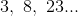What is the sixth term in the sequence?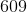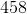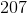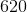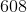Explanation:

The fourth term is: 3(23) – 1 = 69 – 1 = 68.

The fifth term is: 3(68) – 1 = 204 – 1 = 203.

The sixth term is: 3(203) – 1 = 609 – 1 = 608.

### Example Question #2 : How To Find The Nth Term Of An Arithmetic Sequence

Consider the following sequence of numbers: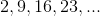What will be the 8th term in the sequence?

58

51

60

56

49

51

Explanation:

Each number in the sequence in 7 more than the number preceding it.

The equation for the terms in an arithmetic sequence is an = a1 + d(n-1), where d is the difference.

The formula for the terms in this sequence is therefore an = 2 + 7(n-1).

Plug in 8 for n to find the 8th term:

a8 = 2 + 7(8-1) = 51

### Example Question #31 : Sequences

Find the seventh term in the following sequence: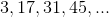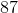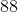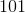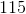Explanation:

The difference between each term can be found through subtraction. For example the difference between the first and the second term can be found as follows: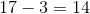One can check and see that this is the case for the other given numbers in the sequence as well.

In order to find the seventh term, expand the sequence by adding 14 to the last given number (4th number) and all of the following numbers until the 7th number in the sequence is reached.

This gives the sequence: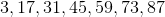As seen above the seventh number in the sequence is 87 and the correct answer.

### Example Question #1 : How To Find The Nth Term Of An Arithmetic Sequence

What is the tenth number in the sequence: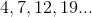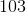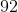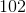Explanation:

The purpose of this question is to understand the patterns of sequences.

First, an equation for the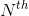term in the sequence must be determined (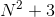).

This is true because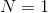will create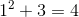,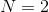will create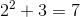,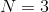will create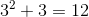,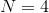will create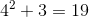.

Then, the eqution must be applied to find the specified term. For the tenth term, the expression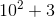must be evaluated, yielding 103.

### Example Question #1 : How To Find The Nth Term Of An Arithmetic Sequence

You are given a sequence with the same difference between consecutive terms. We know it starts atand its 3rd term is. Find its 10th term.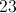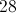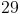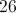Explanation:

From the given information, we know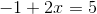, which means each consecutive difference is 3.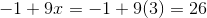### Example Question #10 : How To Find The Nth Term Of An Arithmetic Sequence

An arithmetic sequence begins as follows: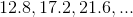Give the sixteenth term of this sequence.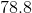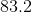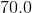None of the other responses give the correct answer.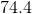Explanation:

Subtract the first term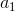from the second term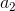to get the common difference: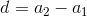Setting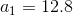and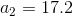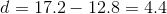Theth term of an arithmetic sequence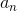can be found by way of the formula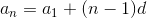Setting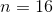, and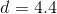in the formula: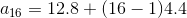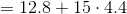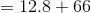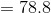← Previous 1

### All SAT Math Resources